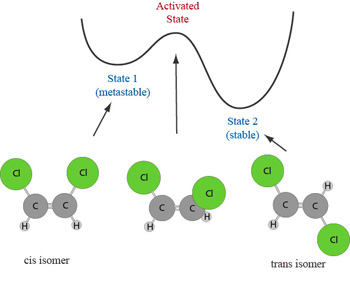# Entropy Activity

-Introduction

-The reaction coordinate

-The energy landscape

-Population distributions

-Motion at constant temperature, and exchange of energy with the heat bath

-Thermally activated processes

-Energy and temperature determine the populations

-Entropy and free energy

-Mathematical derivation of free energy

 < Previous | Next >

## The reaction coordinate

The first feature we will explore is the meaning of the x-axis in the above figure, which is known as the reaction coordinate Q. For a system to get from state 1 to state 2 it must follow some pathway. In most cases, we don't have detailed information on the precise nature of this pathway, but it is nevertheless useful to imagine motion along a single dimension and consider the potential energy of the system as it moves along this path. The potential energy along the reaction coordinate is called the "energy landscape".

One example for which the pathway is easy to envision is the cis-trans isomerization shown below. In this case, Q is the dihedral angle about the double bond. The transition state occurs when the dihedral angle is about 90o. We can consider all angles <90o to correspond to the cis isomer (state 1) and all angles >90o to correspond to the trans isomer (state 2).Our simulations will use a much simpler system, but one that retains the essentials of a thermally activated process. The system is that of the rectangular box demonstrated in lecture.

Virtual Activity

- The reaction coordinate (Java Required) [Opens in a separate window. Please read through all content in the lower window, using the scroll bar.]

 < Previous | Next >